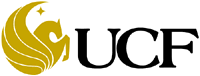Computational GeometryCOT5520 - Computational Geometry

Fall 2003, 3Hrs
M W 16:30 - 17:45pm
Room: Eng II 302
Prof. Amar Mukherjee
Office 208 CSB
Office and/or email hours: 15:30 to 16:30 M W
Email Address: amar@cs.ucf.edu
Telephone: 407-823-2763
Teaching Associate: Khurram Hassan Shafique.
Email: khurram@cs.ucf.edu
Tele: 407-823-4733
Office hours: Tu Th 15:00 to 16:00 hrs CSB-103
Academic Calendar

Final Exam Schedule: Monday December 8, 2003; 16:00 to 18:50

Course Pre-requisite

Pre-requisite: Background in Design and Analysis of Algorithms or Instructor's permission. Seniors are encouraged to take this course.

Course Description and Goals

Computational geometry is the study of algorithms for solving geometric problems on a computer. The field of computational geometry is less than 20 years old and a thriving community of researchers has emerged working on fundamental problems relevant to several application domains including computer graphics, solid modeling, computer generated forces,virtual reality, simulated training, computer-aided ma nufacturing, robotics, computer vision, VLSI design, CAD/CAM, geographic information systems, and statistics.

The goal of the course is to teach the fundamental paradigms for designing efficient algorithms dealing with collections of geometric objects such as points, lines, line segments, planes, or higher dimensional objects. The class assignments will consist of homework problems, a midterm exam, a term project and a final exam.

The major topics include the following:

• Introduction: Classical Geometries; Computational Geometry; DCEL (doubly connected edge list); Preliminary concepts: line,line segment,polygon,simple polygon,convex polygon,kernel,triangulation,planar graph equations,right,left, above,below computation; geometric duality; computational real RAM.
• Convex Hulls: Definition. Graham scan; lower and upper bound; Jarvis's march,Divide and Conquer algorithm; Preparata-Hong algorithm; Mergehull; Dynamic Convex Hull algorithms;
• Geometric Searching Problems: Location problems and Range Search Problems; polygon inclusion; Planar point location problem: slab method; trapezoidal maps, a randomized incremental algorithm; Kirkpatrick's triangle search method. Range search problems: 1-dimensional range searching; K-D tree method; range tree method ,Range tree for d-dimension, fractional cascading
• Proximity Problems: Closest Pair Problem;All Nearest Neighbor Problem; Voronoi Diagram; Properties of Voronoi diagram; Construction of Voronoi Diagram; Fortune's algoritthm, Relationship between Voronoi diagram and other proximity problems; Closest Pair algorithm; Farthest Pair Problem and algorithm.
• Polygon Triangulation: Art Gallery Problem, Triangulating a monotone polygon, partitioning a polygon into monotone pieces; Euclidean minimum spanning tree; Construction of EMST via Delaunay Triangulation; traveling salesman problem.
• Triangulation methods: Delaunay triangulation; greedy algorithm; Other methods; Constrained Triangulation;
• Intersection Problem: line intersection problem: line segment intersection algorithm in two dimensions; intersection of convex polygons; doubly connected edge list, computing the overlay of two subdivisions;intersection of Half Spaces and relation to two-variable linear programming. Rectangle Intersection Problem.
• Additional Topics covered by students' term projects: Arrangements and Duality; Binary Space Partition, Robot Motion Planning, Visibility Graphs, Quadtrees, 3-D Convex Hull

• Reference Text:
• 1. M. de Berg, M. van Kreveld, M. Overmars, O. Schwarzkopf, `` Computational Geometry Algorithms and Applications", Springer-Verlag, 1999.

This all-new introduction to computational geometry is a textbook for high-level undergraduate and low-level graduate courses. The focus is on algorithms and hence the book is well suited for students in computer science and engineering. Motivation is provided from the application areas: all solutions and techniques of computational geometry are related to particular applications in robotics, graphics, CAD/CAM, and geographic information systems. For students this motivation will be especially welcome.

• 2. F.P.Preparata and M.I.Shamos, ``Computational Geometry : An Introduction" Springer-Verlag,1985. This is the classic text on Computational Geometry
• 3. Joseph O'Rourke, ``Computational Geometry in C", Cambridge University Press ( 1995 reprint).

• Methods of Evaluation (Subject to Change)
Homework Assignments 35%; Midterm(s): 20%; Term project: 20%; Final:25%
Grading: >95% is A; 90-95% A-; >85% B+, 82-85% B,80-81% B-
C and D grades are broken down similarly like B in the 70's and 60's percentages. Any score below 60% is F.

• Lectures
• Homework
• Prog.Assign.
• Reading
• Term Project

• Pointers to Related WWW Pages
• Computational Geometry Resourses
• Computational Geometry Web Pages
• Computational Geometry Journals
• Computational Geometry iin Action

• Last modified August, 2003